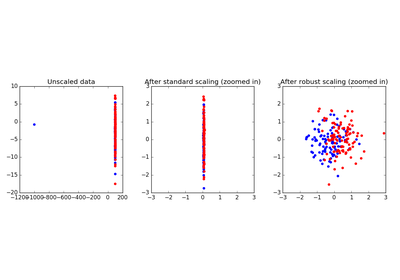# `sklearn.preprocessing`.RobustScaler¶

class `sklearn.preprocessing.``RobustScaler`(with_centering=True, with_scaling=True, quantile_range=(25.0, 75.0), copy=True)[source]

Scale features using statistics that are robust to outliers.

This Scaler removes the median and scales the data according to the quantile range (defaults to IQR: Interquartile Range). The IQR is the range between the 1st quartile (25th quantile) and the 3rd quartile (75th quantile).

Centering and scaling happen independently on each feature (or each sample, depending on the axis argument) by computing the relevant statistics on the samples in the training set. Median and interquartile range are then stored to be used on later data using the transform method.

Standardization of a dataset is a common requirement for many machine learning estimators. Typically this is done by removing the mean and scaling to unit variance. However, outliers can often influence the sample mean / variance in a negative way. In such cases, the median and the interquartile range often give better results.

New in version 0.17.

Read more in the User Guide.

Parameters: with_centering : boolean, True by default If True, center the data before scaling. This does not work (and will raise an exception) when attempted on sparse matrices, because centering them entails building a dense matrix which in common use cases is likely to be too large to fit in memory. with_scaling : boolean, True by default If True, scale the data to interquartile range. quantile_range : tuple (q_min, q_max), 0.0 < q_min < q_max < 100.0 Default: (25.0, 75.0) = (1st quantile, 3rd quantile) = IQR Quantile range used to calculate `scale_`. New in version 0.18. copy : boolean, optional, default is True If False, try to avoid a copy and do inplace scaling instead. This is not guaranteed to always work inplace; e.g. if the data is not a NumPy array or scipy.sparse CSR matrix, a copy may still be returned. center_ : array of floats The median value for each feature in the training set. scale_ : array of floats The (scaled) interquartile range for each feature in the training set. New in version 0.17: scale_ attribute.

`robust_scale`
Equivalent function without the object oriented API.
`sklearn.decomposition.PCA`
Further removes the linear correlation across features with ‘whiten=True’.

Notes

See examples/preprocessing/plot_robust_scaling.py for an example.

Methods

 `fit`(X[, y]) Compute the median and quantiles to be used for scaling. `fit_transform`(X[, y]) Fit to data, then transform it. `get_params`([deep]) Get parameters for this estimator. `inverse_transform`(X) Scale back the data to the original representation `set_params`(\*\*params) Set the parameters of this estimator. `transform`(X[, y]) Center and scale the data
`__init__`(with_centering=True, with_scaling=True, quantile_range=(25.0, 75.0), copy=True)[source]
`fit`(X, y=None)[source]

Compute the median and quantiles to be used for scaling.

Parameters: X : array-like, shape [n_samples, n_features] The data used to compute the median and quantiles used for later scaling along the features axis.
`fit_transform`(X, y=None, **fit_params)[source]

Fit to data, then transform it.

Fits transformer to X and y with optional parameters fit_params and returns a transformed version of X.

Parameters: X : numpy array of shape [n_samples, n_features] Training set. y : numpy array of shape [n_samples] Target values. X_new : numpy array of shape [n_samples, n_features_new] Transformed array.
`get_params`(deep=True)[source]

Get parameters for this estimator.

Parameters: deep : boolean, optional If True, will return the parameters for this estimator and contained subobjects that are estimators. params : mapping of string to any Parameter names mapped to their values.
`inverse_transform`(X)[source]

Scale back the data to the original representation

Parameters: X : array-like The data used to scale along the specified axis.
`set_params`(**params)[source]

Set the parameters of this estimator.

The method works on simple estimators as well as on nested objects (such as pipelines). The latter have parameters of the form `<component>__<parameter>` so that it’s possible to update each component of a nested object.

Returns: self :
`transform`(X, y=None)[source]

Center and scale the data

Parameters: X : array-like The data used to scale along the specified axis.

## Examples using `sklearn.preprocessing.RobustScaler`¶Robust Scaling on Toy Data